# Create an event based on a process¶

This example gives elements to create an event based on a multivariate stochastic process. Let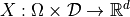be a stochastic process of dimension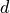, where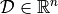is discretized on the mesh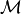. We suppose thatcontains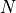vertices.

We define the event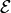as: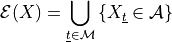where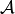is a domain of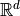.

A particular domainis the cartesian product of type: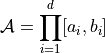In that case, the library definesby its both extreme points :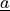and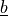.

In the general case,is a Domain object that is able to check if it contains a given point in.

The library creates an Event object from the process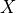and the domain. Then, it is possible to get a realization of the event, which is scalar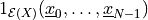if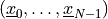is a realization ofon.

import openturns as ot

ot.Log.Show(ot.Log.NONE)


Define a 2-d mesh

indices = [40, 20]
mesher = ot.IntervalMesher(indices)
lowerBound = [0.0, 0.0]
upperBound = [2.0, 1.0]
interval = ot.Interval(lowerBound, upperBound)
mesh = mesher.build(interval)


Create the covariance model

amplitude = [1.0, 2.0, 3.0]
scale = [4.0, 5.0]
spatialCorrelation = ot.CorrelationMatrix(3)
spatialCorrelation[0, 1] = 0.8
spatialCorrelation[0, 2] = 0.6
spatialCorrelation[1, 2] = 0.1
covmodel = ot.ExponentialModel(scale, amplitude, spatialCorrelation)

# Create a normal process
process = ot.GaussianProcess(covmodel, mesh)


Create a domain A in R^3: [0.8; 1.2]*[1.5; 1.6]*[0.5; 0.7]

lowerBound = [0.8, 1.5, 0.5]
upperBound = [1.2, 1.6, 0.7]
domain = ot.Interval(lowerBound, upperBound)

# Create the event
event = ot.ProcessEvent(process, domain)